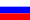# Vestnik КRAUNC. Fiz.-Mat. nauki. 2022. vol. 38. no. 1. P. 40-53. ISSN 2079-6641

Contents of this issue

Read Russian VersionMSC 34B37, 34B15

Research Article

On a nonlocal problem for impulsive differential equations with mixed maxima

T. K. Yuldashev¹²

¹National University of Uzbekistan, 100174, Tashkent, Universitetskaya street, 4, Uzbekistan
²V. I. Romanovskii Institute of Mathematics, Academy of Sciences of Uzbekistan, 100174, Tashkent, Universitetskaya street, 4-B, Uzbekistan

E-mail: tursun.k.yuldashev@gmail.com

A nonlocal boundary value problem for a first order system of ordinary integro-differential equations with impulsive effects and mixed maxima is investigated. The boundary value problem is given by the integral condition. The method of successive approximations in combination it with the method of compressing mapping is used. The existence and uniqueness of the solution of the boundary value problem are proved. The continuous dependence of the solutions on the right-hand side of the boundary condition is showed.

Key words: impulsive integro-differential equations, nonlocal boundary condition, mixed maxima, successive approximations, existence and uniqueness of solution, continuous dependence of solution.

DOI: 10.26117/2079-6641-2022-38-1-40-53

Original article submitted: 02.03.2022

Revision submitted: 21.04.2022

For citation. Yuldashev T. K. On a nonlocal problem for impulsive differential equations with mixed maxima. Vestnik KRAUNC. Fiz.-mat. nauki. 2022, 38: 1, 40-53. DOI: 10.26117/2079-6641-2022-38-1-40-53

Competing interests. The author declares that there are no conflicts of interest with respect to authorship and publication.

Contribution and responsibility. The author contributed to the writing of the article and is solely responsible for submitting the final version of the article to the press. The final version of the manuscript was approved by the author.

References

1. Anguraj A., Arjunan M. M. Existence and uniqueness of mild and classical solutions of impulsive evolution equations, Elect. J. of Differential Equations, 2005. vol. 2005, no. 111, pp. 1–8.
2. Ashyralyev A., Sharifov Y. A. Existence and uniqueness of solutions for nonlinear impulsive differential equations with two–point and integral boundary conditions, Advances in Difference Equations, 2013. vol. 2013, no. 173 DOI: 10.1186/1687-1847-2013-173.
3. Bin L., Xinzhi L., Xiaoxin L. Robust global exponential stability of uncertain impulsive systems, Acta Mathematika Scientia, 2005. vol. 25, no. 1, pp. 161–169.
4. Benchohra M., Henderson J., Ntouyas S. K. Impulsive differential equations and inclusions. Contemporary mathematics and its application. New York: Hindawi Publishing Corporation, 2006.
5. Boichuk A. A., Samoilenko A. M. Generalized inverse operators and Fredholm boundary-value problems (2nd ed.). Berlin — Boston: Walter de Gruyter GmbH, 2016. 314p. pp.
6. Boichuk A. A., Samoilenko A. M. Generalized inverse operators and Fredholm boundary-value problems. Utrecht: Brill, 2004.
7. Ji Sh., Wen Sh. Nonlocal Cauchy problem for impulsive differential equations in Banach spaces, Intern. J. of Nonlinear Science, 2010. vol. 10, no. 1, pp. 88–95.
8. Halanay A., Veksler D. Teoria calitativa a sistemelor cu impulsuri. Bucuresti: Editura Academiei Republicii Socialiste Romania, 1968.
9. Lakshmikantham V., Bainov D. D., Simeonov P. S. Theory of impulsive differential equations. Singapore: World Scientific, 1989. 434 p. pp.
10. Perestyk N. A., Plotnikov V. A., Samoilenko A. M., Skripnik N. V. Differential equations with impulse effect: multivalued right-hand sides with discontinuities. DeGruyter Stud. V.40. Math. Berlin: Walter de Gruter Co., 2011.
11. Samoilenko A. M., Perestyk N. A. Impulsive differential equations. Singapore: World Sci., 1995.
12. Ashyralyev A., Sharifov Y. A. Optimal control problems for impulsive systems with integral boundary conditions, Elect. J. of Differential Equations, 2013. vol. 2013, no. 80, pp. 1–11 DOI: 10.1063/1.4747627.
13. Djamalov S. Z., Ashurov R. R., Turakulov H. Sh.On a semi-nonlocal boundary value problem for the three-dimensional Tricomi equation of an unbounded prismatic domain, Vestnik KRAUNC. Fiz.-Mat. Nauki, 2021. vol. 35, no. 2, pp. 8–16, DOI: 10.26117/2079-6641-2021-35-2-8-16 (In Russian).
14. Li M., Han M. Existence for neutral impulsive functional differential equations with nonlocal conditions, Indagationes Mathematcae, 2009. vol. 20, no. 3, pp. 435–451.
15. Mardanov M. J., Sharifov Y. A., Molaei H. H. Existence and uniqueness of solutions for first-order nonlinear differential equations with two-point and integral boundary conditions, Electr. J. of Differential Equations, 2014. vol. 2014, no. 259, pp. 1–8.
16. Sharifov Ya. A. Optimal control for systems with impulsive actions under nonlocal boundary conditions, Russian Mathematics (Izv. VUZ), 2013. vol. 57, no. 2, pp. 65–72.
17. Sharifov Y. A. Conditions optimality in problems control with systems impulsive differential equations with nonlocal boundary conditions, Ukrainain Math. Journ., 2012. vol. 64, no. 6, pp. 836–847.
18. Sharifov Ya. A. Optimal control problem for systems with impulsive actions under nonlocal boundary conditions, Vestnik Samarskogo Gos. Tekhn. Univ. Seria: Fiziko-matem. nauki, 2013. vol. 33, no. 4, pp. 34–45 (In Russian).
19. Sharifov Y. A., Mammadova N. B. Optimal control problem described by impulsive differential equations with nonlocal boundary conditions, Differential equations, 2014. vol. 50, no. 3, pp. 403–411.
20. Yuldashev T. K. Solvability of a boundary value problem for a differential equation of the Boussinesq type, Differential equations, 2018. vol. 54, no. 10, pp. 1384–1393 DOI: 10.1134/S0012266118100099.
21. Yuldashev T.K. Nonlocal boundary value problem for a nonlinear Fredholm integro-differential equation with degenerate kernel, Differential equations, 2018. vol. 54, no. 12, pp. 1646–1653 10.1134/S0012266118120108.
22. Yuldashev T. K. Nonlocal mixed-value problem for a Boussinesq-type integro-differential equation with degenerate kernel, Ukrainian Mathematical Journal, 2016. vol. 68, no. 8, pp. 1278–1296 DOI: 10.1007/s11253-017-1293-y.
23. Yuldashev T. K. Nonlocal problem for a mixed type differential equation in rectangular domain, Proceedings of the Yerevan state univers. Physical and Mathematical Sciences, 2016. no. 3, pp. 70–78.
24. Yuldashev T. K. Spectral features of the solving of a Fredholm homogeneous integro-differential equation with integral conditions and reflecting deviation, Lobachevskii Journal of Mathematics, 2019. vol. 40, no. 12, pp. 2116–2123 DOI: 10.1134/S1995080219120138.

Yuldashev Tursun Kamaldinovich – D. Sci. (Phys. & Math.), Associate Professor, Professor of the Uzbek-Israel Joint Fac., National University of Uzbekistan, Tashkent, Uzbekistan, ORCID 0000-0002-9346-5362.